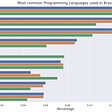# Multiplication Using Machine Learning

`#creating dataframedf = pd.DataFrame()df['first_digit'] = [x for x in range(1,1001)]df['pk'] = 1#crossjoin dataframedf2 = df.merge(df,on='pk',how='outer').drop('pk',axis=1)#renaming columnsdf2.rename(columns={'first_digit_x':'first_digit','first_digit_y':'second_digit'},inplace=True)#Creating target column (first*second)df2['product'] = df2["first_digit"]*df2["second_digit"]`
`X = df2.drop('product',axis=1)y = df2['product']#80-20 split of data-setX_train, X_test, y_train, y_test = train_test_split(X, y, test_size=0.2, random_state=42)`
`%%time#linear-regressionlr_time_start = time.time()lr = LinearRegression()lr.fit(X_train,y_train)lr_time_stop = time.time()print(f"LR Model Trained: {lr_time_stop-lr_time_start}")#random-forestrf_time_start = time.time()rf = RandomForestRegressor()rf.fit(X_train,y_train)rf_time_stop = time.time()print(f"RF Model Trained: {rf_time_stop-rf_time_start}")#lgbmlgbm_time_start = time.time()lgr = LGBMRegressor()lgr.fit(X_train,y_train)lgbm_time_stop = time.time()print(f"LGBM Model Trained: {lgbm_time_stop-lgbm_time_start}")#xgboostxgb_time_start = time.time()xgb = XGBRegressor()xgb.fit(X_train,y_train)xgb_time_stop = time.time()print(f"XGB Model Trained: {xgb_time_stop-xgb_time_start}")`

--

--

--

## More from Himanshu Bhardwaj

I am an Analyst working on building ML driven solution for industry problems.

Love podcasts or audiobooks? Learn on the go with our new app.

## M-LSD : Machine Learning Model for Detecting Wireframes## Using Deep neural networks for mortgage finance## Information Theory Basic for Machine Learning & Deep Learning## Siri, what is an RNN?## Ensemble techniques — Improvement over traditional Decision Trees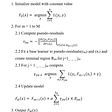## Machine Learning with Google Colab## Learning to Run a Power Network Challenge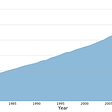## ZeroToGans Course Project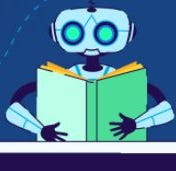## Himanshu Bhardwaj

I am an Analyst working on building ML driven solution for industry problems.

## Part 3 : Percentages, Percentiles and Quartiles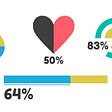## Machine Learning Basics## What are the Most used programming languages and databases in Brazil?